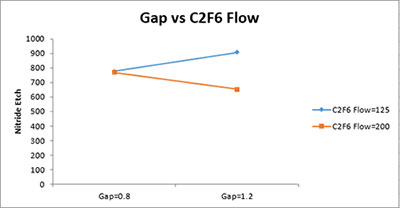# Two Factor Plot WorksheetThis worksheet contains the plots of every pair of factors. An example for one two factor plot is given below. The plot shows the impact each pair of factors has on the response variable. This type of chart provides insights into which interactions might be significant. Interactions might be significant if the lines on the chart are not parallel.